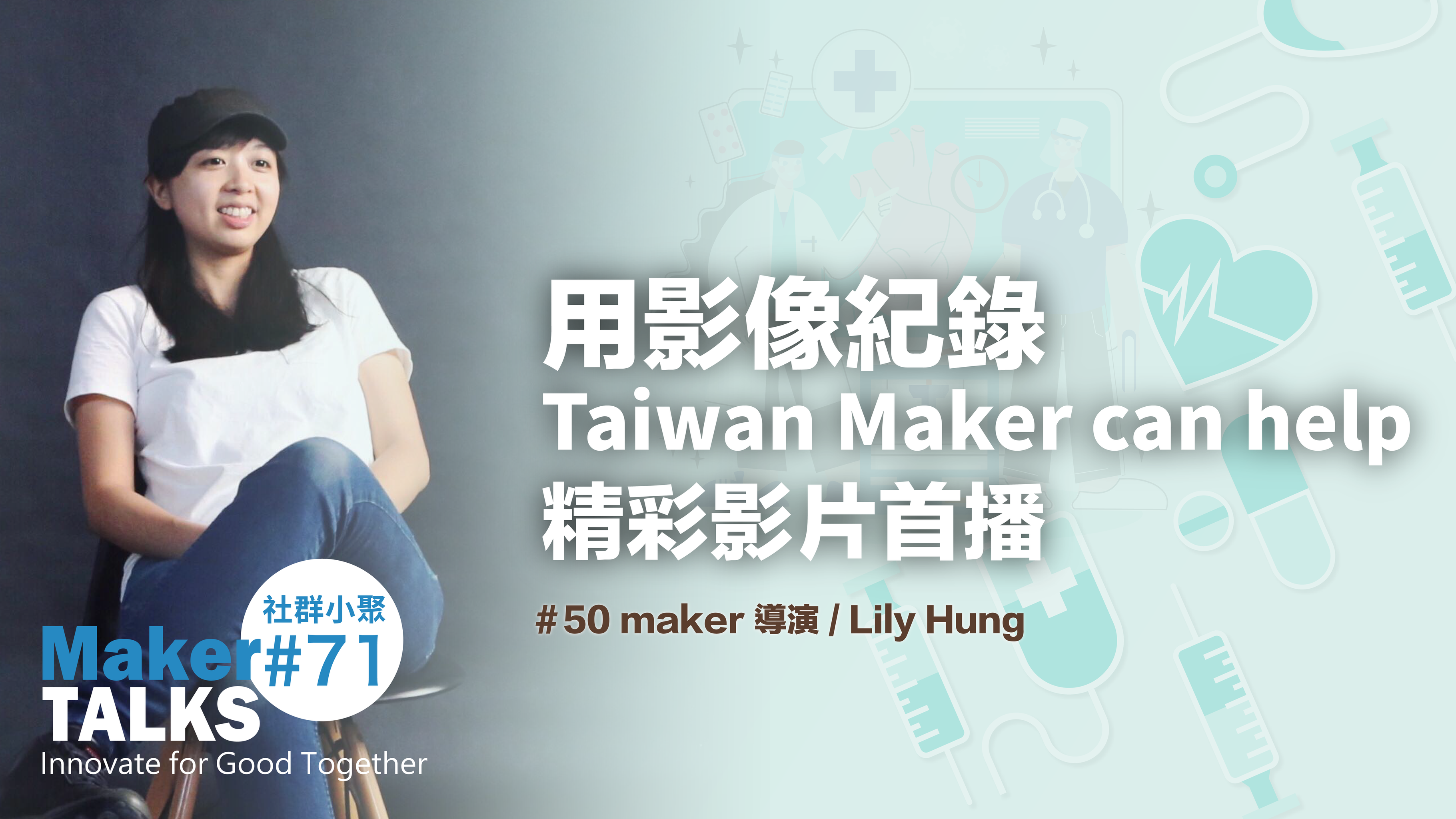# 【CAVEDU講堂】影像辨識技術結合Q1ARM機器手臂進行物品分類

2. 嵌入式(或個人電腦)Python控制核心的程式設計### 一、Q1-ARM的操作概念說明

Q1-ARM是一個具有5個自由度的機器手臂，五個自由度分別是：底座(左右旋轉)、下臂(上下旋轉)、上臂(上下旋轉)、腕部(上下旋轉)、夾爪(開閉旋轉)，基本上都是透過SG-90伺服馬達進行機構的運動，因此無法乘載過重的負載，但用於機器手臂基礎控制教學是非常合用的，相關機器手臂的組裝檔案連結如下：組裝說明Q1-ARM機器手臂

```
123456#include
Servo __myservo16;
Servo __myservo11;
Servo __myservo9;
Servo __myservo8;
Servo __myservo7;

```

```
12345678if (Serial.available()) {
// 讀取傳入的字串直到"\n"結尾

String_to_Int(str, 2);
Serial.println(SA);
Serial.println(A);
}

```

```
12345678910111213141516if (SA == "數值") {
if (A &gt;= PRE_angle) {
for (int angle=PRE_angle;angle&lt;=A;angle++){
__myservo16.write(angle);
delay(20);
}
Serial.println("Servo_OK");
}
if (A &lt; PRE_angle) { for (int angle=PRE_angle;angle&gt;=A;angle--){
__myservo16.write(angle);
delay(20);
}
Serial.println("Servo_OK");
}
}

```

```
1234567891011121314void String_to_Int(String temp, int count)
{
int index;

index = temp.indexOf(',');
SA = temp.substring(0, index);

for (int i = 1; i &lt; count; i++) {
temp = temp.substring(index + 1, temp.length());
index = temp.indexOf(',');
SA[i] = temp.substring(0, index);
A[i] = SA[i].toInt();
}
}

```

### 二、嵌入式系統(或個人電腦)運算操作的概念說明```
123python3 PHOTO_ARM.py --video 攝影機編號 --com UART連接埠編號
#例如
python3 PHOTO_ARM.py --video 0 --com 5

```

```
123python PHOTO_ARM.py --video 攝影機編號 --com UART連接埠編號
#例如
python PHOTO_ARM.py --video 0 --com 5

```LOBE.ai在完成匯入訓練資料集與神經網路模型訓練後，本篇專題教學採用Tensorflow-Lite的模型進行匯出模型應用。1. Webcam攝影機完成連接於嵌入式系統(或個人電腦)，並且確認攝影機連接編號。
2. Q1-ARM完成連接於嵌入式系統(或個人電腦)，並且確認UART串列通訊埠的編號。
3. ai訓練後的神經網路模型資料夾與Python程式存放在同一個資料夾中。

1. UART串列資料傳輸設定
2. Tensorflow-Lite模型匯入
3. 影像分類推論
4. 推論結果控制機器手臂

```
1python3 LOBE_WEBCAM_ARM.py --video 攝影機編號 --com UART連接埠編號 --model "模型資料夾"

```

```
1python3 PHOTO_ARM.py --video 0 --com 5 --model "ARM_MODEL_LOBE TFLite"

```

```
12345Condition_1 = 'OBJ_1'
Condition_2 = 'OBJ_2'
Condition_3 = 'OBJ_3'
Condition_4 = 'OBJ_4'
Condition_Other = 'Other'

```

```
123456def get_prediction(image, interpreter, signature):
影像推論的程式片段(略)
return outputs
#image是即時影像資料
#interpreter是神經網路模型
#signature是模型參數檔內容

```

get_prediction是影像推論的函式

```
12345def process_image(image, input_shape):
#影像前處理的程式片段(略)
return image.reshape(input_shape).astype(np.float32)
#image是即時影像資料
#input_shape是輸入影像的解析度資訊

```

process_image是影像預處理函式

```
123COM_PORT = args.com  #COM_PORT變數設定UART通訊連接埠的編號
BAUD_RATES = 9600  #BAUD_RATES變數設定RAUD為9600BPS
ser = serial.Serial(COM_PORT, BAUD_RATES)  # 建立 ser 物件啟動序列通訊

```
```
12if (times==1) and (key_flag == 0) and (Motion_Busy ==0):
prediction = get_prediction(image, interpreter, signature)

```

```
12345if (classification_flag == 1) and (Label_name == Condition_1) and (prob &gt; 0.5):
Condition_1_count = Condition_1_count + 1
Condition_2_count = 0
Condition_3_count = 0
Condition_4_count = 0

```

```
1234if (Condition_1_count == 50):
Motion_Busy = 1
伺服馬達動作角度設定片段程式(略)
Motion_Busy = 0

```

Condition_1連續穩定辨識次數達50次時開始進行機器手臂伺服馬達的動作設定。 Condition_n，以此類推。
”降低影像辨識推論的運算負荷，times>=10：表示每10個Frame進行一次影像辨識推論。”

```
#進行各種按鍵偵測的動作程式片段(略)
# 按 q鍵 或 Esc鍵 結束程式
key_detect = 1

```

```
12345678if (key_flag == 1):
cv2.rectangle(image_src,
(box_left,box_top),(box_left+scale,box_top+scale),
(0,255,0),2)
elif (key_flag == 0):
cv2.rectangle(image_src,
(box_left,box_top),(box_left+scale,box_top+scale),
(0,0,255),2)

```

```
123456789101112131415161718box_center_x = box_left + int(scale / 2)
box_center_y = box_top + int(scale / 2)

dw = int(frame_width / 2) - box_center_x
dh = frame_height - box_center_y
angle = (math.atan(dw / dh)) / math.pi * 180

cv2.line(image_src,
(int(frame_width / 2),frame_height),
(box_center_x,box_center_y),
(0,255,255),2)

cv2.putText(image_src, str(round(angle,3)),
(int(frame_width * 0.6),30), cv2.FONT_HERSHEY_SIMPLEX, 1,
(0,255,255), 6, cv2.LINE_AA)
cv2.putText(image_src, str(round(angle,3)),
(int(frame_width * 0.6),30), cv2.FONT_HERSHEY_SIMPLEX, 1,
(0,0,0), 2, cv2.LINE_AA)

```

```
1cv2.imshow('Detecting_1 ....',image_src)

```

（本文經CAVEDU同意轉載，原文連結；責任編輯：謝涵如）Author: CAVEDU 教育團隊

CAVEDU 教育團隊是由一群對教育充滿熱情的大孩子所組成的機器人科學教育團隊。致力推動國內機器人教育。